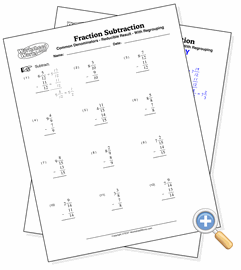# Mixed With Regrouping

## Fraction SubtractionDevelop subtraction skills with mixed fractions requiring regrouping

These problems involve the subtraction of mixed fractions having uncommon denominators which also require regrouping. This is the most complex fraction subtraction problem as it requires both modifying one or more fractions and borrowing from the whole number place for the first fraction.